# OliNo

Renewable Energy

## 40W incandescent lightbulb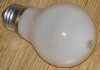This article gives interesting light parameters of an ordinary incandescent lamp of 40 W. It serves as a nice comparison article for the newer type of light bulbs like LED light bulbs.  An Eulumdat file is added in this article.

See this overview for a comparison with other light bulbs.

### Summary measurement data

parameter meas. result remark
Color temperature 2606 K (Deep) warm white
Luminous intensity Iv 31 Cd
Beam angle 339 deg
Power P 42 W
Power Factor 1.0 For every 1 kWh net power consumed, there has been 0.0 kVAhr for reactive power.
Luminous flux 439 lm
Luminous efficacy 10 lm/W
CRI_Ra 99 Color Rendering Index.
Coordinates chromaticity diagram x=0.4697 and y=0.4150
Fitting E27
D x H external dimensions 60 x 106 mm External dimensions of the light bulb.
D x H luminous area 60 x 80 mm Dimensions of the luminous area (used in Eulumdat file). This is equal to the size of the bulb itself (without the screw fitting).
General remarks The ambient temperature during the whole set of measurements was 24-27 deg C.

Warm up effect: the warm up time is 5 minutes and th eilluminance increases with 5 %.

Voltage dependency: there is a linear dependency of  illuminance and power consumption on the voltage.

The Eulumdat file can be found via this link.

This light bulb is measured on dim-abilty, and interesting parameters are put together in one graph, as function of the mechanical dim position.

### Eulumdat light diagram

An interesting graph is the light diagram, indicating the intensity in the C0-C180 and the C90-C270 plane. This light diagram below comes from the program Qlumedit, that extracts these diagrams from an Eulumdat file.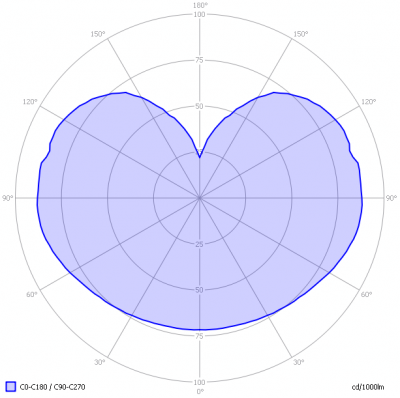The light diagram giving the radiation pattern.

It indicates the luminous intensity around the light bulb. This light diagram is symmetrical around the z-axis.

The radiation sideways is higher than to the front, since the luminous area of the lightbulb sideways is bigger.

The unit is Cd/1000lm, meaning the intensity in Cd assuming there would be 1000 lumen in the measured light bulb. This enables comparing different types of light bulbs.

### Illuminance Ev at 1 m distance, or luminous intensity Iv

Herewith the plot of the averaged luminous intensity Iv as a function of the inclination angle with the light bulb.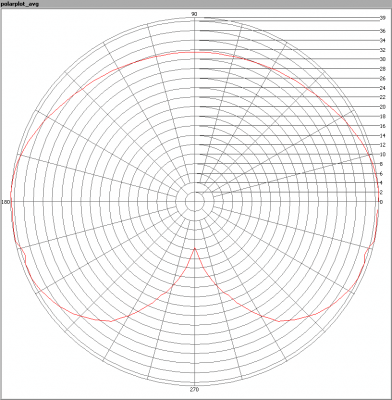The radiation pattern of the light bulb.

This radiation pattern is the same as the one given earlier. This is because in this light bulb’s case, the radiation pattern is symmetric around the z-axis, meaning that the averaged pattern given here is the same as the extraction of the Eulumdat file.

These averaged values are used (later) to compute the lumen output.Intensity data of every measured turn angle at each inclination angle.

This plot shows per inclination angle the intensity measurement results for each turn angle at that inclination angle. So at 90 degrees inclination angle, all the measurement results of illuminance for all measured turn angles are in the range of 106-149 % of the illuminance value at 0 degrees inclination angle.

When using the average values per inclination angle, the beam angle can be computed, being 339 degrees.

### Luminous flux

With the averaged illuminance data at 1 m distance, taken from the graph showing the averaged radiation pattern, it is possible to compute the luminous flux.

The result of this computation for this light spot is a luminous flux of 439 lm.

### Luminous efficacy

The luminous flux being 439 lm, and the power of the lightbulb being 42 W, yields a luminous efficacy of 10 lm/W.

A power factor of 1.0 means that for every 1 kWh net power consumed, a reactive component of 0.1 kVAr was needed.

 Light bulb voltage 230 V Light bulb current 183 mA Power P 42 W Apparent power S 42 VA Power factor 1.0

### Color temperature and Spectral power distribution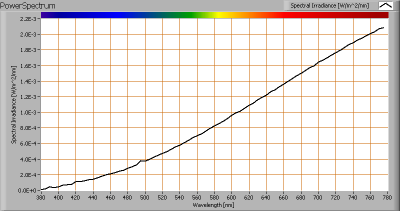The spectral power distribution of this light bulb.

The measured color temperature is about 2600 K which is (deep) warm white.

This color temperature is measured straight underneath the light bulb. Below a graph showing the color temperature for different inclination angles.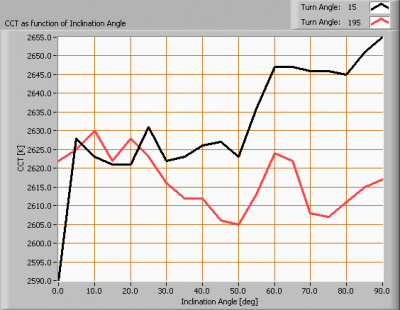Color temperature as a function of inclination angle.

The colortemperature is given for inclination angles up to 90 degrees, no measurements were taken beyond that.

The value remains constant within 1 %.

### Chromaticity diagram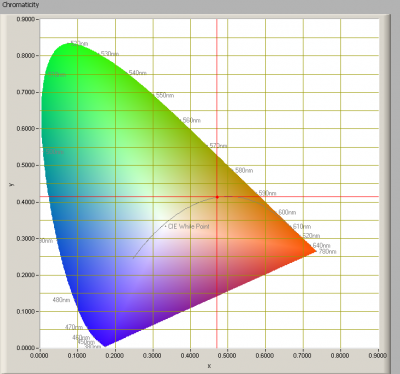The chromaticity space and the position of the lamp’s color coordinates in it.

The light coming from this lamp is on top of the Planckian Locus (the black path in the graph).

Its coordinates are x=0.4697 and y=0.4150.

### Color Rendering Index (CRI) or also Ra

Herewith the image showing the CRI as well as how well different colors are represented (rendered). The higher the number, the better the resemblance with the color when a black body radiator would have been used (the sun, or an incandescent lamp).

Each color has an index Rx, and the first 8 indexes (R1 .. R8) are averaged to compute the Ra which is equivalent to the CRI.CRI of the light2 of this lightbulb.

The value of 99 is higher than 80 which is considered a minimum value for indoor usage.

Note: the chromaticity difference is 0.0009 indicates the distance to the Planckian Locus. Its value is lower than 0.0054, which means that the calculated CRI result is meaningful.

### Voltage dependency

The dependency of a number of lamp parameters on the lamp voltage is determined. For this, the lamp voltage has been varied and its effect on the following lamp parameters measured: illuminance E_v [lx], color temperature CT or correlated color temperature CCT [K], the lamppower P [W] and the luminous efficacy [lm/W].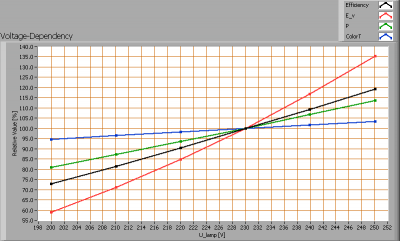Lamp voltage dependencies of certain light bulb parameters, where the value at 230 V is taken as 100 %.

The consumed power and the illuminance vary largely when the voltage varies between 200 – 250 V. This variation is linear.

When the voltage at 230 V varies with + and – 5 V, then the illuminance varies with less than + and – 8 %, which might be visible when the voltage changes abruptly.

### Warm up effects

After switch on of a cold lamp, the effect of heating up of the lamp is measured on illuminance E_v [lx], color temperature CT or correlated color temperature CCT [K], the lamppower P [W] and the luminous efficacy [lm/W].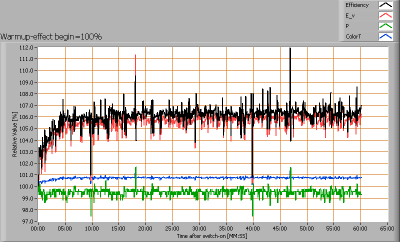Effect of warming up on different light bulb parameters. At top the 100 % level is put at begin, and at bottom at the end.

The illuminance increases about 5 % during the warmup time of 5 minutes.

### Dim-ability

This chapter explains how well this light bulb be dimmed. It is quite common to dim incandescent light bulbs. The dimmer used is a leading edge dimmer.

The measurements taken show Power, Illuminance, Color Temperature and the computed value of Efficacy as function of the mechanical dim position of the dimmer.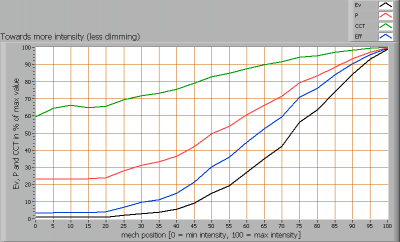Lamp parameters as function of the mechanical position of the dimmer

The value 100 corresponds to minimum dimmig, and the value 0 corresponds to maximum dimming. This can be compared with the extreme mechanical positions of the mechanical knob to adjust the dimming function.

The graphs title is “towards more intensity”  which means that measurements taken started with the light bulb in max dimming position, towards min dimming position. Also the mesurements were done going towards less intensity, and the results were the same fo this lightbulb.

When dimming more and more, the illuminance drops quicker than the power consumption does resulting an a decrease of efficacy.

The color temperature decreases as well with more dimming.